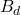# Permeance

## What is Permeance?

Permeance is a measure of the ease with which a magnetic flow is applied using an object or a magnetic circuit. Permeance is the reciprocal of reluctance.

Permeance is directly proportional to the magnetic flux and is denoted by the letter P.

From the above equation we can say that the quantity of magnetic flux for a number of ampere-turns is depended on permeance.

In terms of magnetic permeability, permeance is given byWhere,  = Permeability of free space (vacuum) =  Henry/meter = Relative permeability of a magnetic material = Length of the magnetic path in meter = Cross sectional area in square meters ()

In an electrical circuit, conductance is the level at which an object transmits electricity; similarly, permeance is the degree to which a magnetic flux conducts a magnetic field. Therefore, the permeance is greater in large cross-sections and smaller in smaller cross-sections. This concept of working in a magnetic circuit is similar to operating in electricity c

### Permeance Coefficient

Permeance coefficient is defined as the ratio of magnetic flux density to magnetic field strength at the operating slope of the B-H curve.

It is used to express the “operating point” or “operating slope” of the magnet on the load line or B-H curve. Thus the permeance coefficient is very useful in designing magnetic circuits. It is denoted by PC.

Where,

•= Magnetic flux density at the operating point of B-H curve
•= Magnetic field strength at the operating point of B-H curve

In the above graph, the straight line OP passing between the origin and the B_d and H_d points on the B-H curve (also called as demagnetization curve) is called the permeance line and the slope of the permeance line is the permeance coefficient PC.

For the only single magnet, that is when there is no other permanent magnet (hard magnetic material) or soft magnetic material placed nearby, we can calculate the permeance coefficient PC from the shape and the dimensions of the magnet. Therefore, we can say that the permeance coefficient is a figure of merit for a magnet.Scroll to Top
Scroll to Top